# MCAT Physical : Ions and Isotopes

## Example Questions

### Example Question #1 : Ions And Isotopes

In the operation of nuclear reactors, engineers make use of substances known as neutron poisons. These are used to help store nuclear waste and slow nuclear reactions, but are also generated naturally in nuclear chain reactions as a by-product. This natural by-product can stop the desirable chain reaction present in a nuclear reactor used for power generation.

For example, in nuclear power plants, U-235 is used as a fuel. U-235 absorbs a neutron, and subsequently generates neutrons (which power the chain reaction) and Xe-135. Xe-135 is a well-known neutron poison, and thus can impact the continued chain reaction of a nuclear power plant if it becomes over abundant during power generation.

To help account for this, engineers have developed measurements to quantify the impact of Xe-135 on nuclear operations. For instance, the time during which there is an inability to start a reactor due to the buildup of Xe-135 is referred to as the precluded start-up time. Also, the amount of time that the reactor cannot override the effects of built up Xe-135 is called poison outage time. Perhaps the most important measure that engineers have developed is the neutron absorption capacity (σ), which is measured in units of barns and is a function of microscopic cross section. Xe-135 has a neutron absorption capacity of 2.00 * 106 barns, while another common poison, Sm-149, has a neutron absorption capacity of 74,500 barns.

A scientist studying the reaction above is surprised to find traces of Xe-124 in his analysis of the reaction. How is Xe-124 different from Xe-135?

Xe-135 is a more massive ion than Xe-124

Xe-124 has 11 fewer electrons

Xe-124 has 11 fewer protons

Xe-124 has 11 fewer positrons

Xe-135 is a more massive isotope than Xe-124

Xe-135 is a more massive isotope than Xe-124

Explanation:

Xe-135 and Xe-124 are isotopes of xenon, not ions. Ions have charges, while isotopes have varying atomic mass numbers; thus, Xe-135 is a more massive isotope of xenon than is Xe-124.

Note that this change in mass can only be attributed to neutron numbers. Changing proton number would alter the elemental identity. Changing electron number would not alter the mass, and would create a charge discrepancy.

### Example Question #2 : Ions And Isotopes

The isotope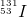has _________ protons, _________ neutrons, and _________ electrons.

78 . . . 78 . . . 53

53 . . . 78 . . . 78

131 . . . 53 . . . 131

53 . . . 131 . . . 53

53 . . . 78 . . . 53

53 . . . 78 . . . 53

Explanation:

The lower number, the atomic number, indicates the number of protons. The upper number, the mass number, indicates the sum of protons and neutrons. The number of neutrons would be equal to the number of protons subtracted from the total mass number. In a neutral atom, the number of protons and electrons is equal in order to balance charge.The atomic number of iodine is 53, meaning there must be 53 protons. The total mass number is 131.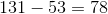The remaining mass must come from neutrons; thus there are 78 neutrons. Since the atom is neutral, the electrons will balance the protons. In total, there are 53 protons, 78 neutrons, and 53 electrons.

### Example Question #1 : Ions And Isotopes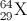Which of the following combination of particles accurately describes the above element?

29 protons, 64 neutrons, 35 electrons

29 protons, 35 neutrons, 29 electrons

29 protons, 64 neutrons, 29 electrons

29 protons, 93 neutrons, 29 electrons

29 protons, 35 neutrons, 35 electrons

29 protons, 35 neutrons, 29 electrons

Explanation:

The lower number next to the atomic symbol gives the number of protons, 29. The upper number gives the number of protons plus neutrons, so the number of neutrons is given by the difference between upper and lower numbers: 64 - 29 = 35. Finally, this is a neutral isotope since no net charge is noted, so the number of electrons must equal the number of protons, 29.

### Example Question #4 : Ions And Isotopes

In the operation of nuclear reactors, engineers make use of substances known as neutron poisons. These are used to help store nuclear waste and slow nuclear reactions, but are also generated naturally in nuclear chain reactions as a by-product. This natural by-product can stop the desirable chain reaction present in a nuclear reactor used for power generation.

For example, in nuclear power plants, U-235 is used as a fuel. U-235 absorbs a neutron, and subsequently generates neutrons (which power the chain reaction) and Xe-135. Xe-135 is a well-known neutron poison, and thus can impact the continued chain reaction of a nuclear power plant if it becomes over abundant during power generation.

To help account for this, engineers have developed measurements to quantify the impact of Xe-135 on nuclear operations. For instance, the time during which there is an inability to start a reactor due to the buildup of Xe-135 is referred to as the precluded start-up time. Also, the amount of time that the reactor cannot override the effects of built up Xe-135 is called poison outage time. Perhaps the most important measure that engineers have developed is the neutron absorption capacity (σ), which is measured in units of barns and is a function of microscopic cross section. Xe-135 has a neutron absorption capacity of 2.00 * 106 barns, while another common poison, Sm-149, has a neutron absorption capacity of 74,500 barns.

A scientist is studying atomic radius within a sample of radioactive flourine sourced from a nuclear reactor similar to the one described in the passage. Which of the following changes is likely to result in the largest atomic radius found in the sample?

Removing an electron from flourine

Removing a neutron from flourine

Explanation:

Adding an electron to flourine results in a flourine anion. Anions generally have larger atomic radii than do their original atomic counterparts, and relative to their cation counterparts. Addition or substraction of neutrons has less of an impact due to their neutral charges and location in the nucelus. Adding a proton would increase the charge in the nucleus, convert flourine to neon, and pull the existing electrons in more tightly, decreasing radius.

### Example Question #5 : Ions And Isotopes

In the operation of nuclear reactors, engineers make use of substances known as neutron poisons. These are used to help store nuclear waste and slow nuclear reactions, but are also generated naturally in nuclear chain reactions as a by-product. This natural by-product can stop the desirable chain reaction present in a nuclear reactor used for power generation.

For example, in nuclear power plants, U-235 is used as a fuel. U-235 absorbs a neutron, and subsequently generates neutrons (which power the chain reaction) and Xe-135. Xe-135 is a well-known neutron poison, and thus can impact the continued chain reaction of a nuclear power plant if it becomes over abundant during power generation.

To help account for this, engineers have developed measurements to quantify the impact of Xe-135 on nuclear operations. For instance, the time during which there is an inability to start a reactor due to the buildup of Xe-135 is referred to as the precluded start-up time. Also, the amount of time that the reactor cannot override the effects of built up Xe-135 is called poison outage time. Perhaps the most important measure that engineers have developed is the neutron absorption capacity (σ), which is measured in units of barns and is a function of microscopic cross section. Xe-135 has a neutron absorption capacity of 2.00 * 106 barns, while another common poison, Sm-149, has a neutron absorption capacity of 74,500 barns.

How is a nuclear reaction, as described in the passage, different from a chemcial reaction?

Nuclear reactions frequently involve changes in atomic number; chemical reactions do not

Chemical reactions frequently involve changes in atomic number; nuclear reactions do not

Chemical reactions result in an increase in the entropy of the universe; nuclear reactions do not

Nuclear reactions involve the release and absorption of energy; chemical reactions do not

Nuclear reactions frequently involve changes in electric charge; chemical reactions do not

Nuclear reactions frequently involve changes in atomic number; chemical reactions do not

Explanation:

Nuclear reactions are unique because they can involve the change of one atom to another, by changing the atomic number. Chemical reactions, by and large, keep atomic number constant, but often vary the ionic state of atoms. In this way, chemical reactions can be thought of as generally involving electrons, while nuclear reactions generally involve protons and neutrons.

### Example Question #1 : Ions And Isotopes

An isotope of an element __________.

1. has all the chemical characteristics of the element
2. can be stable, or non-radioactive
3. is designated by the atomic number of the element
4. contributes to the atomic mass of the element, as shown on the periodic table, in proportion to its abundance
5. all of the above

5

2

3

4

1

5

Explanation:

The correct answer is 5. An isotope is a form of an element with a different number of neutrons in the nucleus. Because the proton number (atomic number) is the same, the electron configuration is the same and therefore the atom behaves chemically in an identical manner to other atoms of the same element. Many isotopes are stable, and indeed that is how elements such as chlorine come to have average atomic masses in between integers; chlorine at 35.45 g/mole is a weighted average of the two stable isotopes, chlorine-35 and chlorine-37. Unstable isotopes of any element undergo decay at various rates. Unless they persist in some quantifiable amount, they aren’t really “there” to participate in the weighted average of an atomic mass.

### Example Question #1 : Ions And Isotopes

Which of the following atoms has the same number of neutrons as an atom of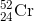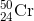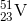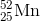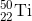Explanation:

In order to find the number of neutrons in an atom when givien isotopic notation, simply subtract the bottom number (atomic number or number of protons) from the top number (mass number or number of protons and neutrons). Doing this with the question atom reveals that it contains 28 neutrons. The only other atom that has this amount is.

### Example Question #8 : Ions And Isotopes

Suppose 0.5 moles of each of the following atoms is dissolved in 500 mL of water:  calcium, oxygen, chlorine, barium, and silver. Which of the following resulting reactions is possible?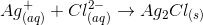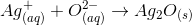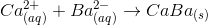Although it appears that one must know solubility rules to answer this question, that is not the case. All but one of the answer choices employs incorrect charges. The periodic table can be used to predict charges of common ions. They are as follows: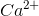,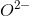,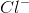,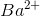, and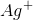. Next, recognize that an ionic compound is formed from a cation (positive) and an anion (negative), and that the total positive charge must equal the total negative charge for a neutral compound. That leaves the reaction between silver and oxygen as the only possible choice.